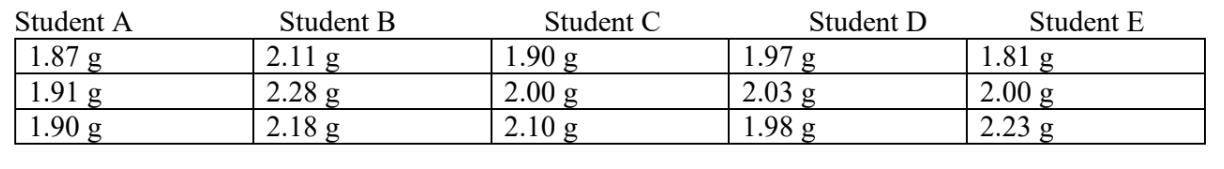# Problem: Five students reported their results from an experiment to remove iron fillings (with a magnet) from a mixture with sand. Each student was given exactly 2.00 grams of sand plus some iron. The table below summarizes the recovered mass of sand. Which student was the most accurate and precise?a. Student Ab. Student Bc. Student Cd. Student De. Student E

###### FREE Expert Solution

We’re being asked to identify the student that is most precise and accurate.

Recall that:

Accuracy refers to how close the measurement is to the ‘true/actual value’.

Precision refers to how close the measurements are to each other.

96% (2 ratings)###### Problem Details

Five students reported their results from an experiment to remove iron fillings (with a magnet) from a mixture with sand. Each student was given exactly 2.00 grams of sand plus some iron. The table below summarizes the recovered mass of sand. Which student was the most accurate and precise?a. Student A
b. Student B
c. Student C
d. Student D
e. Student E